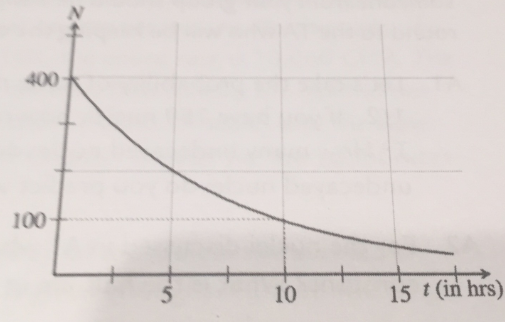# Problem: The curve to the right shows the radioactive decay of a particular sample of a nucleus called Element A. A particular nucleus survives for the first five hours.(a) What's the probability that particular nucleus of Element A will decal between hours 5 and 10? (b) What is the probability that particular nucleus of element A will decay between 5 and 20 hours?

###### FREE Expert Solution

Probability is:

$\overline{){\mathbf{P}}{\mathbf{=}}\frac{{\mathbf{N}}_{\mathbf{0}}\mathbf{-}\mathbf{N}}{{\mathbf{N}}_{\mathbf{0}}}}$

93% (69 ratings)###### Problem Details

The curve to the right shows the radioactive decay of a particular sample of a nucleus called Element A. A particular nucleus survives for the first five hours.

(a) What's the probability that particular nucleus of Element A will decal between hours 5 and 10?

(b) What is the probability that particular nucleus of element A will decay between 5 and 20 hours?